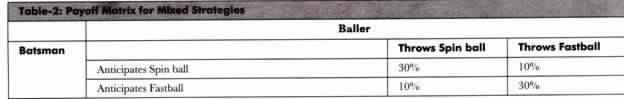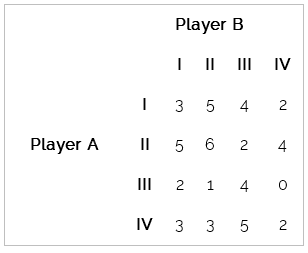# Game Theory Pure and Mixed Strategies, Principle of Dominance

### Pure strategy

A pure strategy is an unconditional, defined choice that a person makes in a situation or game. For example, in the game of Rock-Paper-Scissors,if a player would choose to only play scissors for each and every independent trial, regardless of the other player’s strategy, choosing scissors would be the player’s pure strategy. The probability for choosing scissors equal to 1 and all other options (paper and rock) is chosen with the probability of 0. The set of all options (i.e. rock, paper, and scissors) available in this game is known as the strategy set.

### Mixed strategy

A mixed strategy is an assignment of probability to all choices in the strategy set. Using the example of Rock-Paper-Scissors, if a person’s probability of employing each pure strategy is equal, then the probability distribution of the strategy set would be 1/3 for each option, or approximately 33%. In other words, a person using a mixed strategy incorporates more than one pure strategy into a game.

The definition of a mixed strategy does not rule out the possibility for an option(s)to never be chosen (eg. pscissors= 0.5, prock = 0.5, ppaper = 0). This means that in a way, a pure strategy can also be considered a mixed strategy at its extreme, with a binary probability assignment (setting one option to 1 and all others equal to 0). For this article, we shall say that pure strategies are not mixed strategies.

In the game of tennis, each point is a zero-sum game with two players (one being the server S, and the other being the returner R). In this scenario, assume each player has two strategies (forehand F, and backhand B). Observe the following hypothetical in the payoff matrix:The strategies FS or BS are observed for the server when the ball is served to the side of the service box closest to the returner’s forehand or backhand, respectively. For the returner, the strategies FR and BR are observed when the returner moves to the forehand or backhand side to return the serve, respectively. This gives us the payoffs when the returner receives the serve correctly (FS,FR or BS,BR), or incorrectly (FS,BR or BS,FR). The payoffs to each player for every action are given in pure strategy payoffs, as each player is only guaranteed their payoff given the opponent’s strategy is employed 100% of the time. Given these pure strategy payoffs, we can calculate the mixed strategy payoffs by figuring out the probability each strategy is chosen by each player.

So you are Roger. It is apparent to you that a pure strategy would be exploitable. If you serve to the backhand 100% of the time, it would be easy for the opponent to catch on and return from the backhand side more often than the forehand, maximizing his expected payoff. Same goes for the serve to the forehand. But how often should you mix your strategy and serve to each side to minimize your opponent’s chances of winning? Calculating these probabilities would give us our mixed strategy Nash equilibria, or the probabilities that each strategy is used which would minimize the opponent’s expected payoff. In the following article, we will look at how to find mixed strategy Nash equilibria, and how to interpret them.

### Pure and Mixed Strategies:

In a pure strategy, players adopt a strategy that provides the best payoffs. In other words, a pure strategy is the one that provides maximum profit or the best outcome to players. Therefore, it is regarded as the best strategy for every player of the game. In the previously cited example (Table-1), the increase in the prices of organizations’ products is the best strategy for both of them.

This is because if both of them increase the prices of their products, they would earn maximum profits. However, if only one of the organization increases the prices of its products, then it would incur losses. In such a case, an increase in prices is regarded as a pure strategy for organizations ABC and XYZ.

On the other hand, in a mixed strategy, players adopt different strategies to get the possible outcome. For example, in cricket a bowler cannot throw the same type of ball every time because it makes the batsman aware about the type of ball. In such a case, the batsman may make more runs.

However, if the bowler throws the ball differently every time, then it may make the batsman puzzled about the type of ball, he would be getting the next time.

Therefore, strategies adopted by the bowler and the batsman would be mixed strategies, which are shown ion Table-2:In Table-2, when the batsman’s expectation and the bowler’s ball type are same, then the percentage of making runs by batsman would be 30%. However, when the expectation of the batsman is different from the type of ball he gets, the percentage of making runs would reduce to 10%. In case, the bowler or the batsman uses a pure strategy, then any one of them may suffer a loss.

Therefore, it is preferred that bowler or batsman should adopt a mixed strategy in this case. For example, the bowler throws a spin ball and fastball with a 50-50 combination and the batsman predicts the 50-50 combination of the spin and fast ball. In such a case, the average hit of runs by batsman would be equal to 20%.

This is because all the four payoffs become 25% and the average of four combinations can be derived as follows:

0.25(30%) + 0.25(10%) + 0.25(30%) + 0.25(10%) = 20%

However, it may be possible that when the bowler is throwing a 50-50 combination of spin ball and fastball, the batsman may not be able to predict the right type of ball every time. This would decrease his average run rate below 20%. Similarly, if the bowler throws the ball with a 60-40 combination of fast and spin ball respectively, and the batsman would expect either a fastball or a spin ball randomly. In such a case, the average of the batsman hits remains 20%.

The probabilities of four outcomes now become:

Anticipated fastball and fastball thrown: 0.50*0.60 = 0.30

Anticipated fastball and spin ball thrown: 0.50*0.40 = 0.20

Anticipated spin ball and spin ball thrown: 0.50*0.60 = 0.30

Anticipated spin ball and fastball thrown: 0.50*0.40 = 0.20

When we multiply the probabilities with the payoffs given in Table-2, we get

0.30(30%) + 0.20(10%) + 0.20(30%) + 0.30(10%) = 20%

This shows that the outcome does not depends on the combination of fastball and spin ball, but it depends on the prediction of the batsman that he can get any type of ball from the bowler.

### Principle of Dominance

The principle of dominance in Game Theory (also known as dominant strategy or dominance method) states that if one strategy of a player dominates over the other strategy in all conditions then the later strategy can be ignored.

A strategy dominates over the other only if it is preferable over other in all conditions. The concept of dominance is especially useful for the evaluation of two-person zero-sum games where a saddle point does not exist.

## Dominant Strategy Rules (Dominance Principle)

• If all the elements of a column (say ith column) are greater than or equal to the corresponding elements of any other column (say jth column), then the ith column is dominated by the jthcolumn and can be deleted from the matrix.
• If all the elements of a row (say ith row) are less than or equal to the corresponding elements of any other row (say jth row), then the ith row is dominated by the jth row and can be deleted from the matrix.

### Dominance Example: Game TheoryUse the principle of dominance to solve this problem.

Solution.

 Player B Player A I II III IV Minimum I 3 5 4 2 2 II 5 6 2 4 2 III 2 1 4 0 0 IV 3 3 5 2 2 Maximum 5 6 5 4

There is no saddle point in this game.

#### Using Dominance Property In Game Theory

If a column is greater than another column (compare corresponding elements), then delete that column.
Here, I and II column are greater than the IV column. So, player B has no incentive in using his I and II course of action.

 Player B Player A III IV I 4 2 II 2 4 III 4 0 IV 5 2

If a row is smaller than another row (compare corresponding elements), then delete that row.
Here, I and III row are smaller than IV row. So, player A has no incentive in using his I and III course of action.

 Player B Player A III IV II 2 4 IV 5 2

## 2 thoughts on “Game Theory Pure and Mixed Strategies, Principle of Dominance”

error: Content is protected !!